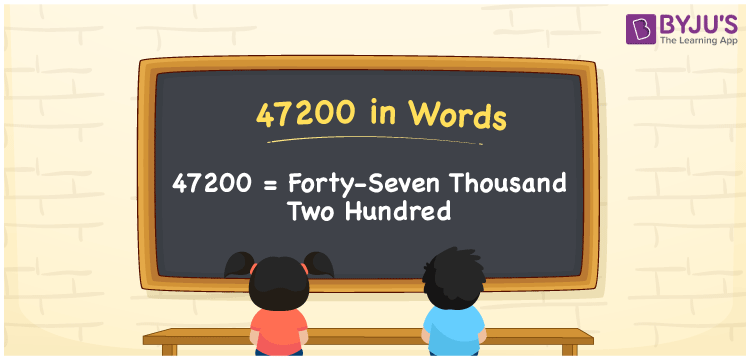# 47200 in Words

47200 in words can be written as Forty-seven Thousand Two Hundred. 47200 indicates a value or a count. If you buy a new refrigerator for Rs. 47200, then you can say that “I purchased a new refrigerator worth Forty-seven Thousand Two Hundred rupees”. In this article, you will learn the conversion of the number 47200 into words in a fun way. Generally numbers in words are written with the help of the English alphabet. So, 47200 in English can be read as “Forty-seven Thousand Two Hundred”.

 47200 in words Forty-seven Thousand Two Hundred Forty-seven Thousand Two Hundred in Numbers 47200

## 47200 in English Words## How to Write 47200 in Words?

Below, you will learn how the number 47200 can be written in words. There are five digits in the number 47200, so let us prepare a place value chart which shows the place value for all the four digits.

 Ten thousands Thousands Hundreds Tens Ones 4 7 2 0 0

We can write it in expanded form as:

4 × Ten thousand + 7 × Thousand + 2 × Hundred + 0 × Ten + 0 × One

= 4 × 10000 + 7 × 1000  + 2 × 100 + 0 × 10 + 0 × 1

= 40000 + 7000 + 200

= 47200

= Forty-seven Thousand Two Hundred

Therefore, 47200 in words is written as Forty-seven Thousand Two Hundred.

47200 is a natural number that precedes 47201 and succeeds 47199.

47200 in words – Forty-seven Thousand Two Hundred

Is 47200 an odd number? – No

Is 47200 an even number? – Yes

Is 47200 a perfect square number? – No

Is 47200 a perfect cube number? – No

Is 47200 a prime number? – No

Is 47200 a composite number? – Yes

## Frequently Asked Questions on 250000 in Words

Q1

### How to write 47200 in words?

47200 in English is written as “Forty-seven Thousand Two Hundred”.
Q2

### Is 47200 an odd number or even number?

47200 is an even number because it is wholly divisible by 2.
47200/2 = 23600
Q3

### Write Forty-seven Thousand Two Hundred in numbers.

Forty-seven Thousand Two Hundred in numbers is 47200.